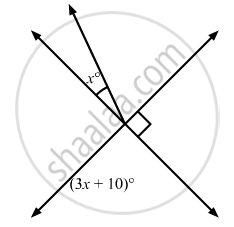# In the Given Figure, the Value of X is - Mathematics

MCQ

In the given figure, the value of x is•  12

•  15

•  20

•  30

#### Solution

The figure is as follows:It is given that

∠2 = 90°

Also,

∠1 = (3X + 10)°       (vertically opposite angles)

Since, x°, ∠1and ∠2form a linear pair.

Therefore,

X +  ∠1 +  ∠2 = 180°

X + (3x + 10° ) + 90°  = 180°

4x + 100°  = 180°

4x = 180°  -100°

4x = 80°

x = (80° )/4

x = 20°

Concept: Concept of Parallel Lines
Is there an error in this question or solution?

#### APPEARS IN

RD Sharma Mathematics for Class 9
Chapter 10 Lines and Angles
Q 8 | Page 52